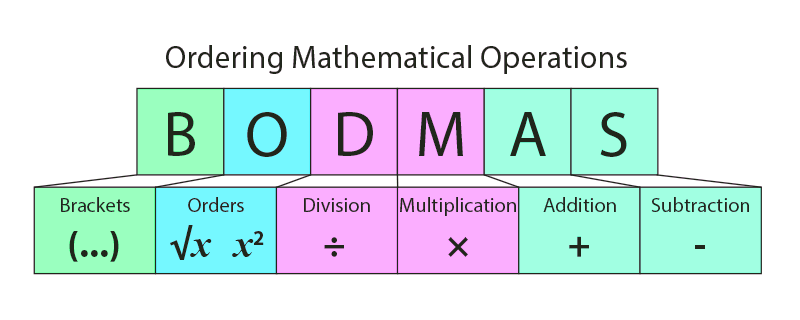# GMAT Math – BODMAS and PEDMAS RulesGMAT Quant Syllabus Consists of –

What is PEMDAS and BODMAS rule?

PEMDAS and BODMAS, these are two different solving techniques based on, in which country you live. BODMAS is the UK based problem-solving technique, whereas, PEMDAS is the US based method.

BODMAS and PEMDAS, both give you a priority sequence of solving an expression. BODMAS stands for-

B          Brackets

O          Index or Order

D         Division

M         Multiplication

S          Subtraction

Whereas, PEMDAS is the abbreviation for

P          Parenthesis

E          Exponents

M         Multiplication

D         Division

S          Subtraction

The only difference between these two methods is, in BODMAS you solve division prior to multiplication, in an equation. While in PEMDAS, the opposite is the case. However, PEDMAS is the synonym of BODMAS.

Here, we will look at a few examples that will refresh the idea for you.

1. Evaluate this expression:

58 ( 2 +  ( 89 ( 112 – 82 ) ) )

Solving this question using the priority of BODMAS, we get;

• First, the innermost bracket will be solved.

58 ( 2 + ( 89 * 30 ) ) // solving the innermost parenthesis

• 58 ( 2 + 2670 ) // Solving the next brackets, subsequently
• 58 ( 2672 ) // Multiplying to get the final result
• 154976

PEMDAS and BODMAS are two similar rules and generate same answer as division and multiplication hold the same weightage.

1. 9900 – 68 of (214 -105) + 72 × 89
• 9900 – 68 of 109 + 72 × 89 // Simplifying ‘bracket’ 214 -105;
• 9900 – 68 * 109 +72 * 89 // Simplifying ‘of’
• 9900 – 7412 + 72 * 89 // Simplifying ‘ multiplication’ 68 * 109
• 9900 – 7412 + 6408 // Simplifying ‘ multiplication’ 72 * 89
• 2488 + 6408           // Simplifying ‘ subtraction’ 9900 – 7412
• 8896 // Simplifying ‘ addition’ 2488 + 6408

We’ll be glad to help you in your GMAT preparation journey. You can ask for any assistance related to GMAT and MBA from us by calling us at +918884544444. You can write to us at gmat@byjus.com.